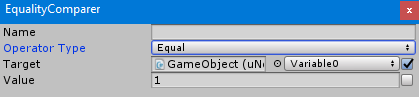# EqualityComparerEqualityComparer is used to compare an object to another object.

There are 6 operator type to chose:

• Equal used to compare if “Target” and “Value” is equal
• Not Equal used to compare if “Target” and “Value” is not equal
• Less Than used to compare if “Target” is less than “Value”
• Greater Than used to compare if “Target” is greater than “Value”
• Less Than Or Equal used to compare if “Target” is less than or equal “Value”
• Greater Than Or Equal used to compare if “Target” is greater than or equal “Value”